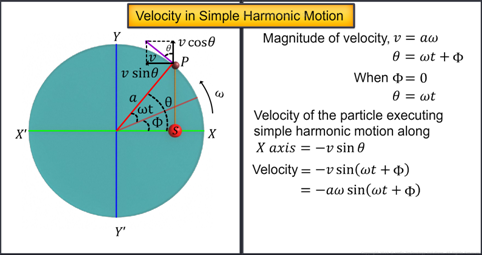Notes On Velocity and Acceleration in SHM - CBSE Class 11 Physics
As you know, when a reference particle revolves along the reference circle with a constant angular velocity, omega, the projection of the particle on the diameters along the X-axis and Y-axis executes simple harmonic motion.The particle executes circular motion with a constant velocity ‘V’ whose magnitude is equal to ‘aω’ where ‘a’ is the radius of the reference circle.   For simplicity, if we assume the initial phase (is equal to zero, then the two components of velocity V are V cos θ and V sin θ respectively in the directions shown.     Then, the component V sin θ represents the velocity of the projection, which is executing simple harmonic motion on the diameter along the X- axis.   Velocity of a particle in simple harmonic motion is equal to - V sin θ.  The negative sign is due to the direction of the velocity at that instant which is opposite to that of the positive X-axis.   If the particle has an initial phase (,  the velocity of simple harmonic motion can be written asOrAcceleration in simple harmonic motionAcceleration of the revolving particle will be the centripetal accelerationThis centripetal acceleration has two components:along the X-axis andalong the Y-axis.   If the initial phase Ф is equal to zero, the acceleration of the projection which is executing simple harmonic motion along the diameter along the X-axis is  equal tosince this component is directed opposite to the positive X-axis.   If the initial phase phi is not equal to zero, the acceleration of the projection in simple harmonic motion is equal toThis can be written as, which in turn can be written asOn rearranging, we getThe quantity a cos omega t plus phi is the displacement x of the particle executing simple harmonic motion.   Hence, the acceleration of the particle executing simple harmonic motion can be written in terms of its displacement as#### Summary

As you know, when a reference particle revolves along the reference circle with a constant angular velocity, omega, the projection of the particle on the diameters along the X-axis and Y-axis executes simple harmonic motion.The particle executes circular motion with a constant velocity ‘V’ whose magnitude is equal to ‘aω’ where ‘a’ is the radius of the reference circle.   For simplicity, if we assume the initial phase (is equal to zero, then the two components of velocity V are V cos θ and V sin θ respectively in the directions shown.     Then, the component V sin θ represents the velocity of the projection, which is executing simple harmonic motion on the diameter along the X- axis.   Velocity of a particle in simple harmonic motion is equal to - V sin θ.  The negative sign is due to the direction of the velocity at that instant which is opposite to that of the positive X-axis.   If the particle has an initial phase (,  the velocity of simple harmonic motion can be written asOrAcceleration in simple harmonic motionAcceleration of the revolving particle will be the centripetal accelerationThis centripetal acceleration has two components:along the X-axis andalong the Y-axis.   If the initial phase Ф is equal to zero, the acceleration of the projection which is executing simple harmonic motion along the diameter along the X-axis is  equal tosince this component is directed opposite to the positive X-axis.   If the initial phase phi is not equal to zero, the acceleration of the projection in simple harmonic motion is equal toThis can be written as, which in turn can be written asOn rearranging, we getThe quantity a cos omega t plus phi is the displacement x of the particle executing simple harmonic motion.   Hence, the acceleration of the particle executing simple harmonic motion can be written in terms of its displacement asPrevious
Next